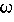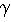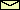# The Linearized Equations of Motion

The equation of motion of the pendulum is nonlinear because of the term02sin. Driving the suspension point leads to a driving force which is also nonlinear in the angle. For small angles, the nonlinear terms can be linearized, i.e., sin=+ O(3) and cos= 1 + O(2).

Thus the linearized equations of motion read

 (horizontal motion) d2dt2 +d/dt +02= a cos 2ft,

 (vertical motion) d2/dt2 +d/dt + (02 + a cos 2ft )= 0,

and

 (rotation) d2/ddt2 +d/dt + (02 + a cos 2ft )= a sin 2ft.

 QUESTION worth to think about: What are the equations of motion linearized around= 180°?
© 1998 Franz-Josef Elmer,Franz-Josef doht Elmer aht unibas doht ch, last modified Sunday, July 19, 1998.